GeoNames Forum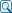Search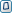Recent Topics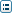Back to home page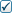Register /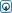LoginAuthor Message
Greg

Joined: 04/06/2018 19:53:43
Messages: 1
Offline

I have written JAVA code to get the nearest big city from geonames API. This code computes a bounding box given a gps location and a distance in order to size the bounding box that is needed by the citiesJSON API call.

You can find the entire project that uses this code at github:

https://github.com/gjkendall/FusionTableModifyJava[url]

The made module of interest is: FusionTableSample.java .

The function BoundingBox uses a close estimate to compute a longitude Ratio for getting east and west bounding box decimal coordinates. It can be off a mile or two depending on how close to the north /south poles you are working with.

The code of interest is class BigCity and class BoundingBox, the code of each is shown here:

class BigCity // BigCityFromLoc
{
public long population;
public String cityName;
public String state;

public BigCity(String location, float km) throws ParseException
{
//json = "{\"lng\":-106.3069722,\"geonameId\":5476825,\"countrycode\":\"US\",\"name\":\"Los Alamos\",\"fclName\":\"city, village,...\",\"toponymName\":\"Los Alamos\",\"fcodeName\":\"seat of a second-order administrative division\",\"wikipedia\":\"en.wikipedia.org/wiki/Los_Alamos%2C_New_Mexico\",\"lat\":35.8880796,\"fcl\":\"P\",\"population\":12019,\"fcode\":\"PPLA2\"}";

BoundingBox bx = new BoundingBox(location, km); // gps coord,km from location

String url = "http://api.geonames.org/citiesJSON?" +
"north=" + bx.north + "&south=" + bx.south + "&east=" + bx.east + "&west=" + bx.west +

String jsonString = FusionTablesSample.getURL(url);
//System.out.println("JSON: "+output);

JSONObject obj = new JSONObject(jsonString);
JSONArray arr = obj.getJSONArray("geonames");

String city = arr.getJSONObject(0).getString("name");
long pop = arr.getJSONObject(0).getLong("population");

Double biglat = arr.getJSONObject(0).getDouble("lat");
Double biglng = arr.getJSONObject(0).getDouble("lng");
String bigloc = biglat.toString()+", "+biglng.toString();
String st = FusionTablesSample.getStateFrmLoc(bigloc); // need the state too for this location.

population = pop;
cityName = city;
state = st;

}
}

// Compute bounding Box coordinates for use with Geonames API.
class BoundingBox
{
public double north, south, east, west;
public BoundingBox(String location, float km)
{
//System.out.println(location + " : "+ km);
String[] parts = location.replaceAll("\\s","").split(","); //remove spaces and split on ,

double lat = Double.parseDouble(parts);
double lng = Double.parseDouble(parts);

double adjust = .008983112; // 1km in degrees at equator.
//adjust = 0.008983152770714983; // 1km in degrees at equator.

//System.out.println("deg: "+(1.0/40075.017)*360.0);

north = lat + ( km * adjust);
south = lat - ( km * adjust);

double lngRatio = 1/Math.cos(Math.toRadians(lat)); //ratio for lng size
//System.out.println("lngRatio: "+lngRatio);

east = lng + (km * adjust) * lngRatio;
west = lng - (km * adjust) * lngRatio;
}

}saphir

Joined: 05/06/2010 22:44:39
Messages: 130
Offline

I do not use Java, hopefully I read it right.

On an ellipsoid earth, the distance for one degree of latitude varies from the equator to the poles, from 110.57 km to 111.69 km.
The different latitude circles’ length depends on this variable distances (to calculate the width of the bounding box).
Calculating a bounding box on an ellipsoid earth is possible, but it would be a mathematical overkill. You rightly use a spherical earth.

However, the base of your calculations is the earth’s circumference of 40075.017 km.
This is the equatorial circumference of the ellipsoid earth, while the corresponding circumference over the poles is only 40007.89 km.

Take an often-used earth radius of 6371 km for your spherical earth, which gives a circumference of 40030 km.

Maybe the errors near the poles will disappear…Go to:  Select a forum   Forum in English ------------- General Administrative Divisions FAQ - frequently asked questions Postal Codes Discussion of GeoNames Toponyms   Forum in other language ------------- Forum in Deutsch